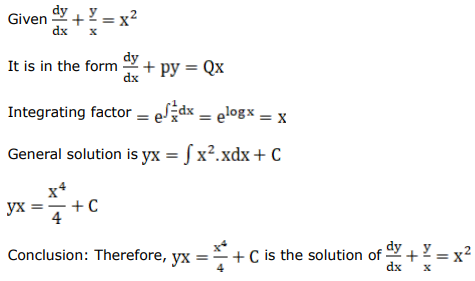# Mark against the correct answer in the following:Question:

Mark $(\sqrt{)}$ against the correct answer in the following:

The general solution of the $D E \frac{d y}{d x}+\frac{y}{x}=x^{2}$ is

A. $x y=x^{4}+\mathrm{C}$

B. $4 x y=x^{4}+C$

C. $3 x y=x^{3}+\mathrm{C}$

D. None of these

Solution: